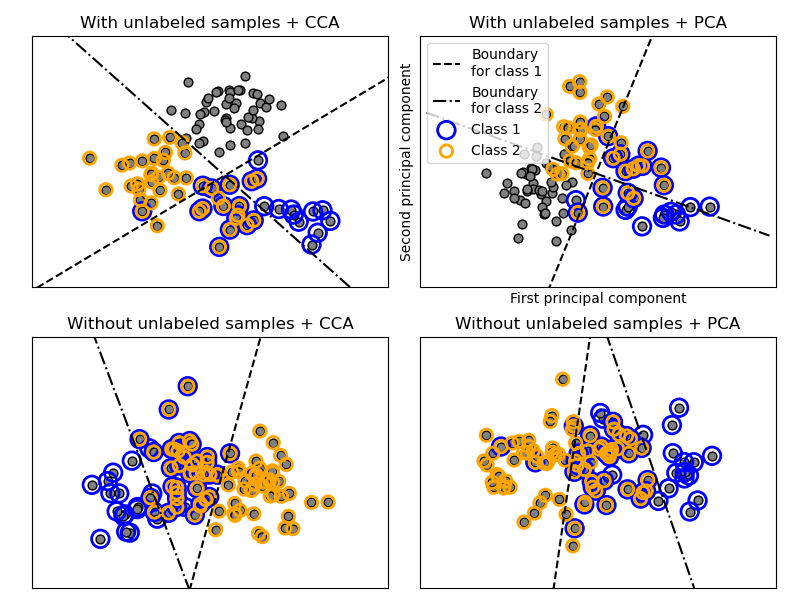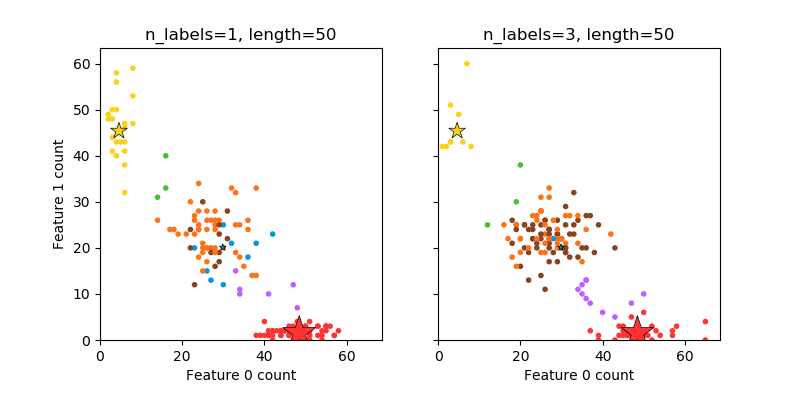# sklearn.datasets.make_multilabel_classification¶

sklearn.datasets.make_multilabel_classification(n_samples=100, n_features=20, *, n_classes=5, n_labels=2, length=50, allow_unlabeled=True, sparse=False, return_indicator='dense', return_distributions=False, random_state=None)

[源码]

• 选择标签数：n〜Poisson（n_labels）
• n次，选择一个类c：c〜多项式（theta）
• 选择文档长度：k〜泊松（长度）
• k次，选择一个单词：w〜多项式（theta_c）

n_samples nt, optional (default=100)

n_features int, optional (default=20)

n_classes int，可选（默认值= 5）

n_labels int, optional (default=2)

length int, optional (default=50)

allow_unlabeled bool, optional (default=True)

sparse bool, optional (default=False)

0.17版中的新功能：允许稀疏输出的参数。
return_indicator ‘dense’ (default),‘sparse’,False

return_distributions bool, optional (default=False)

random_state int, RandomState instance, default=None

X array of shape [n_samples, n_features]

Y array or sparse CSR matrix of shape [n_samples, n_classes]

p_c array, shape [n_classes]

p_w_c array, shape [n_features, n_classes]

## sklearn.datasets.make_multilabel_classification使用示例¶